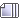###Author Topic: Grumpy's Algebraic therory  (Read 5887 times)

#### willhideho

Hey, what's the square route (or is it root?) of -1 Deoxy?

#### Sheezy

Hey, what's the square route (or is it root?) of -1 Deoxy?
<3

it would be i

#### willhideho

<3

it would be i

Yep. That's right. :D

#### Nightmare-Duckie

9x - 7i > 9x-21

-7i > -21

7i > 21

i > 3

I believe I forgot some stuff about inequalities, so don't punch my snake if I'm wrong please.
/snakepunch
its

i < 3u

Edit: my algebra teacher showed it to us last valentines day. if its on the interwebs anywhere blame him not me...

#### DrMobius

2x=x
2=1
That is a simple way to break algebra. 2=1 is of course the result of dividing x from both equations to determine where they are equal.  2=1 only holds true if x=0.

#### fireball

x=y
x=x
1=1
Dividing by x wasn't what really broke the problem though. Dividing by x is normally perfectly legal unless it has been forced to equal 0.

2x=x
2=1
It only broke by dividing by x there because I already divided by x-y a few steps prior which can only equal 0. It changed the graph of the line from y=x [an infinite number of solutions] to a single point [Only one solution, (0,0)] and since x can now only equal 0 at that step, dividing by 0 a second time only breaks it even more and changes it again from one solution to no solution.

#### Deoxy99

Are you answering the problem? That problem is not algebra! It is a puzzle I made up!

Okay...you are observing a box that looks like it is 75% off the building but not tilting! You run up to fix it! And now you see it moving! Why could that be?
I know what algebra is! x+y=z

#### Sheezy

I know what algebra is! x+y=z
what are x, y, and z supposed to represent though
unless your doing some type of odd 3d graph that makes no sense.

ITT: Morons.

#### Deoxy99

what are x, y, and z supposed to represent though

That was an example. You did not need to solve it!